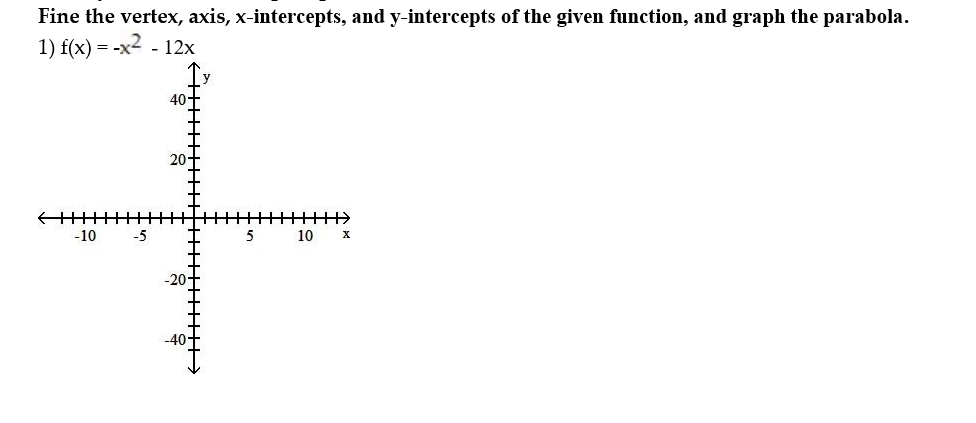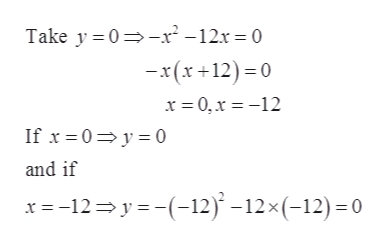# Fine the vertex, axis, x-intercepts, and y-intercepts of the given function, and graph the parabola1) f(x) -x2 -12x40-T20.-10-510-40

Question
5 viewshelp_outlineImage TranscriptioncloseFine the vertex, axis, x-intercepts, and y-intercepts of the given function, and graph the parabola 1) f(x) -x2 -12x 40-T 20. -10 -5 10 -40 fullscreen
check_circle

Step 1

The given function,

Step 2

This is the equation of the parabola.

Find the vertex of the parabola in the following.

Since, the vertex of the parabola = (h, k) in the equation

Step 3

Therefore, the vertex = (-6, 36)

Find the x- intercep...help_outlineImage TranscriptioncloseTake y 0 r-12x 0 -x(x12)0 x 0,x =-12 If x0y0 and if x -12y-12) -12x (-12)0 fullscreen

### Want to see the full answer?

See Solution

#### Want to see this answer and more?

Solutions are written by subject experts who are available 24/7. Questions are typically answered within 1 hour.*

See Solution
*Response times may vary by subject and question.
Tagged in

### Math